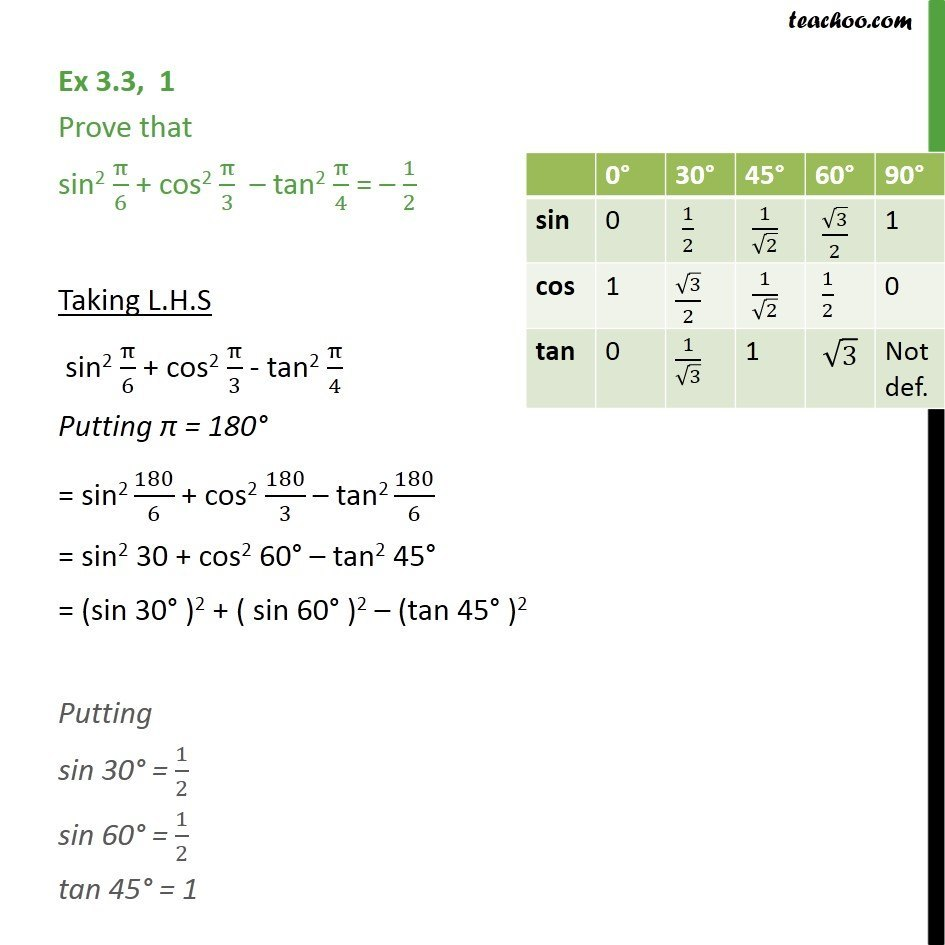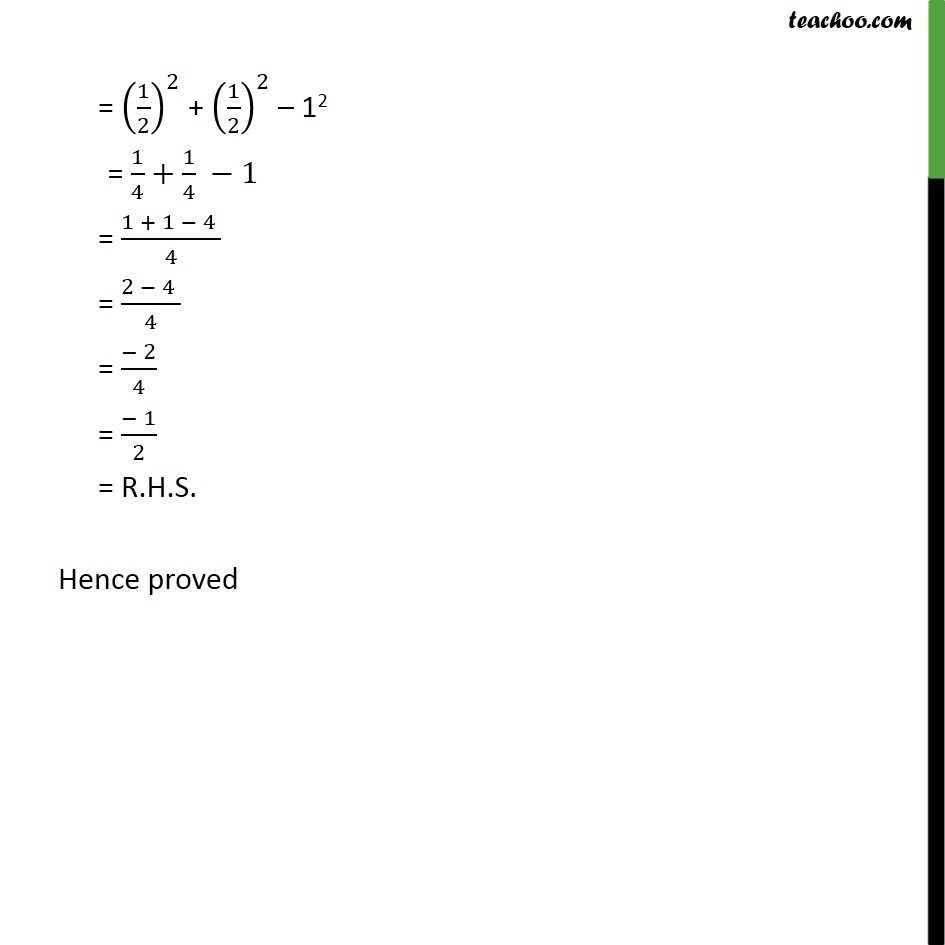Learn All Concepts of Chapter 2 Class 11 Relations and Function - FREE. Check - Trigonometry Class 11 - All Concepts1. Chapter 3 Class 11 Trigonometric Functions
2. Concept wise
3. Finding Value of trignometric functions, given angle

Transcript

Ex 3.3, 1 Prove that sin2 /6 + cos2 /3 tan2 /4 = 1/2 Taking L.H.S sin2 /6 + cos2 /3 - tan2 /4 Putting = 180 = sin2 180/6 + cos2 180/3 tan2 180/6 = sin2 30 + cos2 60 tan2 45 = (sin 30 )2 + ( sin 60 )2 (tan 45 )2 Putting sin 30 = 1/2 sin 60 = 1/2 tan 45 = 1 = (1/2)^2 + (1/2)^2 12 = 1/4+ 1/4 1 = (1 + 1 4 )/4 = (2 4 )/4 = ( 2)/4 = ( 1)/2 = R.H.S. Hence proved

Finding Value of trignometric functions, given angle

About the AuthorDavneet Singh
Davneet Singh is a graduate from Indian Institute of Technology, Kanpur. He has been teaching from the past 9 years. He provides courses for Maths and Science at Teachoo.